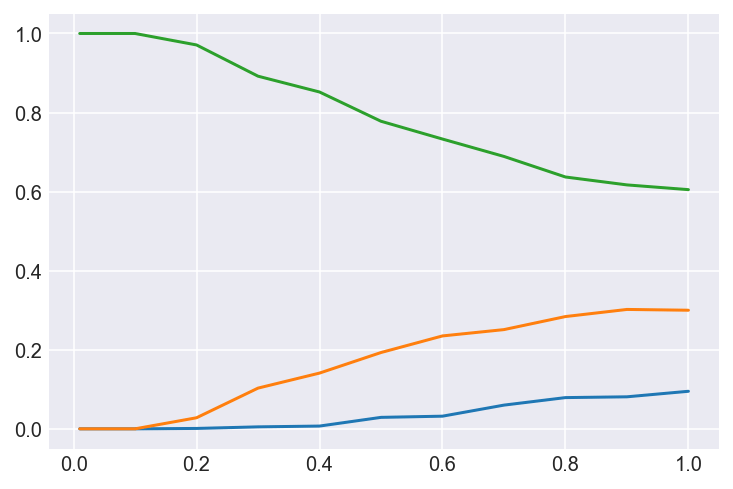September 20, 2021

# How to sample from softmax with temperature

Here is how to sample from a softmax probability vector at different temperatures.

```import numpy as np import matplotlib.pyplot as plt import matplotlib as mpl import seaborn as sns   mpl.rcParams['figure.dpi']= 144   trials = 1000 softmax = [0.1, 0.3, 0.6]   def sample(softmax, temperature): EPSILON = 10e-16 # to avoid taking the log of zero #print(preds) (np.array(softmax) + EPSILON).astype('float64') preds = np.log(softmax) / temperature #print(preds) exp_preds = np.exp(preds) #print(exp_preds) preds = exp_preds / np.sum(exp_preds) #print(preds) probas = np.random.multinomial(1, preds, 1) return probas   temperatures = [(t or 1) / 100 for t in range(0, 101, 10)] probas = [ np.asarray([sample(softmax, t) for _ in range(trials)]).sum(axis=0) / trials for t in temperatures ]   sns.set_style("darkgrid") plt.plot(temperatures, probas) plt.show()```

Notice how the probabilities change at different temperatures. The softmax probabilities are [0.1, 0.3, 0.6]. At the lowest temperatures of 0.01, the dominant index (value 0.6) has near 100% probability of being sampled. At higher temperatures, the selection probabilities move towards the softmax values, e.g. 60% probability for the third index.#### kostas

View all posts by kostas →

This site uses Akismet to reduce spam. Learn how your comment data is processed.